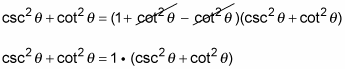##### Trigonometry Workbook For DummiesYou’ll know that you need to factor a trig identity when powers of a particular function or repeats of that same function are in all the terms on one side of the identity.

For example, the expression sin4θ + 2sin2θcos2θ + cos4θ has three terms that you can factor, because they’re the result of squaring a binomial. The pattern you need is the algebraic equivalence a2 + 2ab + b2 = (a + b)2.

1. Factor the expression sin4θ + 2sin2θcos2θ + cos4θ as the square of a binomial.

(sin2θ + cos2θ)2 = 1

2. Now just replace the expression in the parentheses with its equivalent by using the Pythagorean identity.

(1)2 = 1

The preceding example was really simple — as long as you recognized the pattern. It’d be another thing altogether if you went off on some tangent (pardon the pun).

In the next example, the factoring occurs in the numerator of the fraction, where powers of sin x appear. Solve the identity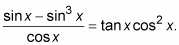1. Factor sin x out of each term in the numerator.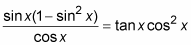2. Replace the expression in the parentheses with its equivalent by using the Pythagorean identity.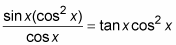3. Now split up the fraction into a product of two fractions, carefully arranging the numerator and denominator.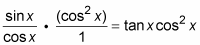4. Replace the first fraction with tan x by using the ratio identity.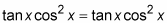This last example requires factoring by using the difference between two squares. The pattern here is the algebraic equation a2 – b2 = (a – b)(a + b) or a4 – b4 = (a2 – b2)(a2 + b2). Solve the identity csc2θ + cot2θ = csc4θ – cot4θ.

1. Factor the two terms on the right by using the difference-of-squares equation.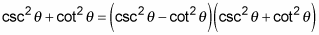2. In the left set of parentheses only, replace the csc2θ with its equivalent in the Pythagorean identity.

You want to keep the two terms in the right parentheses.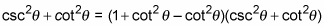3. Now simplify the expression, getting rid of the two opposites.